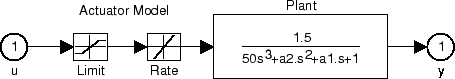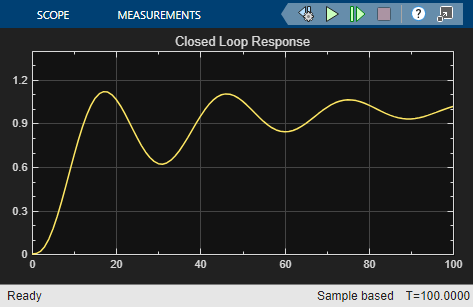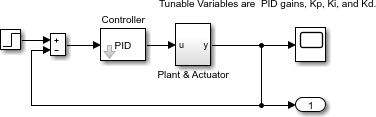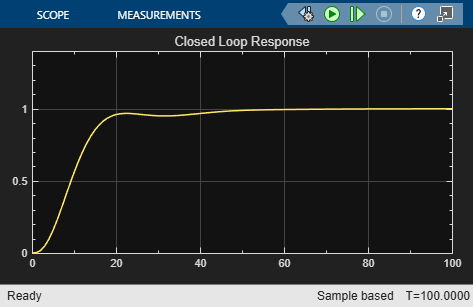# Using `fminimax` with a Simulink Model

This example shows how to tune the parameters of a Simulink® model. The model, `optsim`, is included when you run this example. The model includes a nonlinear process plant modeled as a Simulink block diagram.Plant with Actuator Saturation

The plant is an under-damped third-order model with actuator limits. The actuator limits are a saturation limit and a slew rate limit. The actuator saturation limit cuts off input values greater than 2 units or less than –2 units. The slew rate limit of the actuator is 0.8 units/sec. The closed-loop response of the system to a step input is shown in Closed-Loop Response. You can see this response by opening the model (type `optsim` at the command line or click the model name), and selecting Run from the Simulation menu. The response plots to the scope.Closed-Loop Response

The problem is to design a feedback control loop that tracks a unit step input to the system. The closed-loop plant is entered in terms of the blocks where the plant and actuator are located in a hierarchical Subsystem block. A Scope block displays output trajectories during the design process.Closed-Loop Model

To optimize this system, minimize the maximum value of the output at any time $t$ between 0 and 100. (In contrast, in the example lsqnonlin with a Simulink Model, the solution involves minimizing the error between the output and the input signal.)

The code for this example is contained in the helper function `runtrackmm` at the end of this example. The objective function is simply the output `yout` returned by the `sim` command. But minimizing the maximum output at all time steps might force the output to be far below unity for some time steps. To keep the output above 0.95 after the first 20 seconds, the constraint function `trackmmcon` contains the constraint `yout >= 0.95` from `t = 20` to `t = 100`. Because constraints must be in the form $g\le 0$, the constraint in the function is `g = -yout(20:100) + 0.95`.

Both `trackmmobj` and `trackmmcon` use the result yout from `sim`, calculated from the current PID values. To avoid calling the simulation twice, `runtrackmm` has nested functions so that the value of `yout` is shared between the objective and constraint functions. The simulation is called only when the current point changes.

Call `runtrackmm`.

`[Kp,Ki,Kd] = runtrackmm`
``` Objective Max Line search Directional Iter F-count value constraint steplength derivative Procedure 0 5 0 1.11982 1 11 1.184 0.07978 1 0.482 2 17 1.012 0.04285 1 -0.236 3 23 0.9995 0.007058 1 -0.0186 Hessian modified twice 4 29 0.9997 9.708e-07 1 0.00716 Hessian modified Local minimum possible. Constraints satisfied. fminimax stopped because the size of the current search direction is less than twice the value of the step size tolerance and constraints are satisfied to within the value of the constraint tolerance. ```
```Kp = 0.5910 ```
```Ki = 0.0606 ```
```Kd = 5.5383 ```

The last value in the `Objective value` column of the output shows that the maximum value for all the time steps is just under 1. The closed-loop response with this result is shown in the figure Closed-Loop Response Using fminimax.

This solution differs from the solution obtained in lsqnonlin with a Simulink Model because you are solving different problem formulations.Closed-Loop Response Using `fminimax`

### Helper Function

The following code creates the `runtrackmm` helper function.

```function [Kp, Ki, Kd] = runtrackmm optsim % initialize Simulink(R) pid0 = [0.63 0.0504 1.9688]; % a1, a2, yout are shared with TRACKMMOBJ and TRACKMMCON a1 = 3; a2 = 43; % Initialize plant variables in model yout = []; % Give yout an initial value pold = []; % tracks last pid opt = simset('solver','ode5','SrcWorkspace','Current'); options = optimset('Display','iter',... 'TolX',0.001,'TolFun',0.001); pid = fminimax(@trackmmobj,pid0,[],[],[],[],[],[],... @trackmmcon,options); Kp = pid(1); Ki = pid(2); Kd = pid(3); function F = trackmmobj(pid) % Track the output of optsim to a signal of 1. % Variables a1 and a2 are shared with RUNTRACKMM. % Variable yout is shared with RUNTRACKMM and % RUNTRACKMMCON. updateIfNeeded(pid) F = yout; end function [c,ceq] = trackmmcon(pid) % Track the output of optsim to a signal of 1. % Variable yout is shared with RUNTRACKMM and % TRACKMMOBJ updateIfNeeded(pid) c = -yout(20:100)+.95; ceq=[]; end function updateIfNeeded(pid) if ~isequal(pid,pold) % compute only if needed Kp = pid(1); Ki = pid(2); Kd = pid(3); [~,~,yout] = sim('optsim',[0 100],opt); pold = pid; end end end```